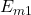## Consider a circular hoop, a disk, and a sphere all having the same mass and radius. These objects all start at rest at the top of a ramp and

Question

Consider a circular hoop, a disk, and a sphere all having the same mass and radius. These objects all start at rest at the top of a ramp and are released to roll down the ramp at the same instant. If they all roll straight down the ramp without slipping or colliding, which one reaches the bottom first?

in progress 0
2 weeks 2021-08-28T18:33:10+00:00 1 Answers 0 views 0

we see that the sphere is the body that arrives with the highest speed, therefore it must be the body that takes the least time,

Explanation:

Let’s analyze this exercise they ask us which body reaches the bottom of the ramp first, for which we must calculate the speed of the body in the final part, use the concepts of energy conservation

starting point. Highest part of the ramp= ​​U = m g h

at this point there is no kinematic energy because the body starts from rest

Final point. Lower part of the ramp

E_{m2} = K = ½ m v² + ½ I w²

we fix the reference system at this point, as the body is rolling, it has energy of connection and rotation

energy is conserved

E_{m1} = E_{m2}²

mgh = ½ m v² + ½ I w²

the angular and linear variables are related

v = wr

w = v / r

mg h = ½ m v² + ½ I v² / r²

mg h = ½ m v² (1 + I / m r²)

v² = 2gh / (1 + I / mr²)

Since all bodies start from rest, the body that has more speed at the end of the ramp, is the body that takes less time.

To find the speed we look in the tables for the moment of inertia of the bodies

circular loop    I = mr²

disk                   I = ½ m r²

sphere              I = 2/5 mr²

let’s calculate the speed for each body

tie

v² = 2g h (1+ mr² / mr²)

v = √ (2gh) / √2

v = 0.707 ra (2gh

disk

v² = 2gh / (1 + ½ mr² / mr²)

v = ra 2gh / ra 3/2

v = 0.816 ra 2gh

sphere

v² = 2gh / (1 + 2/5 mr₂ / mr)

v = ra 2gh / ra 7/5

v = 0.845 ra 2gh

we see that the sphere is the body that arrives with the highest speed, therefore it must be the body that takes the least time, since

t = d / v